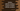# Python infinity : infinite numbers and how to check infinite numbers## Python infinite/infinity numbers Introduction :

Python mathematical module math provides different mathematical constants and functions. One constant is for defining infinity. In this post, I will explain to you about constants and functions used for infinity. With

### Positive and negative python infinity :

inf constant is provided for defining infinity. math.inf is for positive infinity and -math.inf is for negative infinity. It is a floating-point value.

Note that infinity was added in Python 3.5 and it will throw one error for lower python versions.

For the below program :

``````import math

print(math.inf)
print(-math.inf)``````

It will print the below output :

``````inf
-inf``````

Python _math _module also provides methods to check if a number is infinite or not. Following are the two methods that we can use for that :

### math.isfinite(x) :

This method returns one boolean value based on the number x is infinite or NaN. It returns True if the value of x is neither infinity nor a NaN. Otherwise, it returns False.

Let me show you with an example how it works :

``````import math

values = [10, 0.0, -1, math.nan, math.inf, -math.inf]

for item in values:
print(math.isfinite(item))``````

math.nan is a floating-point NaN or Not a number. It is equivalent to float(‘nan’).

This program will print :

``````True
True
True
False
False
False``````

Using this method, there is no way you can find out if a number is NaN or inf. For that, we need to use the below method.

### How to check python infinity using math.isinf(x) :

math.isinf() method can be used to check if a number is infinite or not. It returns True, if the value of x is positive or negative infinity. Else, it returns False.

Let’s use the same list of items we used in the above example :

``````import math

values = [10, 0.0, -1, math.nan, math.inf, -math.inf]

for item in values:
print(math.isinf(item))``````

It will print :

``````False
False
False
False
True
True``````

The last two are True. So, this method is better to find out if a value is infinite or anything else.

__ View on Github